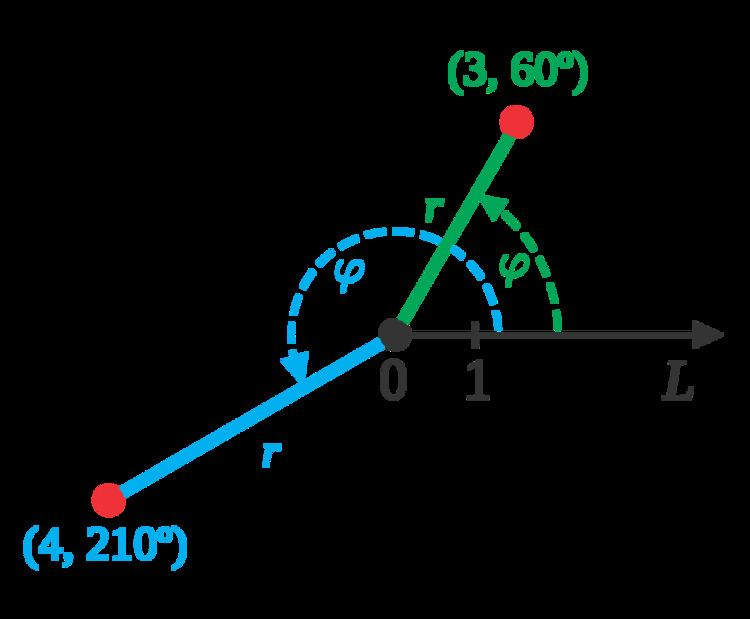# Angle of rotation

Updated on
Covid-19In mathematics, the angle of rotation is a measurement of the amount, the angle, by which a figure is rotated counterclockwise about a fixed point, often the center of a circle. A clockwise rotation is considered a negative rotation, so that for instance a rotation of 310° (counterclockwise) can also be called a rotation of –50° (since 310° + 50° = 360°, a full rotation). A counterclockwise rotation of more than one complete turn is normally measured modulo 360°, meaning that 360° is subtracted off as many times as possible to leave a non-negative measurement less than 360°.

For example, the carts on a Ferris wheel move along a circle around the center point of that circle. If a cart moves around the wheel once, the angle of rotation is 360 degrees. If the cart was stuck halfway, at the top of the wheel, at that point its angle of rotation was only 180 degrees.

This is also referred to as the "order of symmetry."

Angles are commonly measured in degrees, radians, gons (gradians) and turns, sometimes also in angular mils and binary radians. They are central to polar coordinates and trigonometry.

## References

Similar Topics
Aliens of the Deep
Philip Morehead
Shiraz Shivji
Topics

 B i Link H2
 L